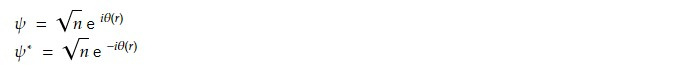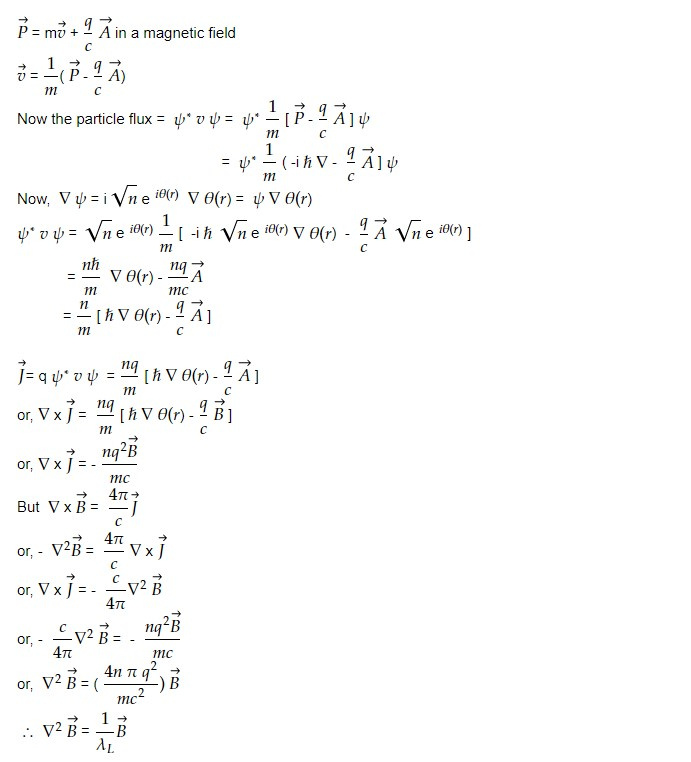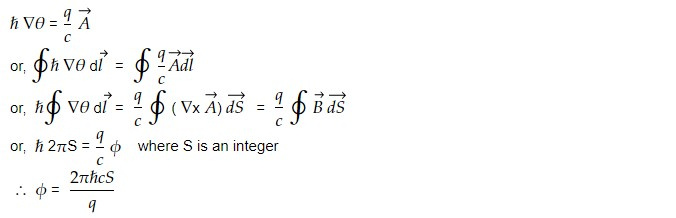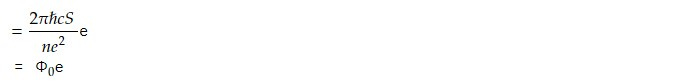# Flux quantization in a superconducting ring

Flux quantization is a fundamental property of superconducting rings, which is a consequence of the Meissner effect. When a superconducting ring is placed in a magnetic field, the magnetic field is excluded from the interior of the ring due to the Meissner effect. This means that the flux through the ring, which is given by the product of the magnetic field and the area of the ring, is quantized in units of the magnetic flux quantum.

For a cooper pair, if n be the concentration, the wave function can be written asAnd we know that the canonical momentum isIt shows that the Boson gas obeys London's equation.

But Meissner effect says that B and J both should be zero deep inside and superconductor so from above equation ,Hence flux inside a super conductor ring (Φ) is quantized as integral multiple of 2𝜋ℏcS/q.

We can rewrite the expression 2𝜋ℏcS/q in terms of fundamental constants:

2𝜋ℏcS/q = 2𝜋ℏcS/(ne)where Φo is the magnetic flux quantum and Φ = nΦo.

Therefore, the expression 2𝜋ℏcS/q represents the magnetic flux quantum in units of e, the elementary charge, which is equivalent to the magnetic flux quantum divided by e.

Flux quantization is a fundamental property of superconductors that has several important implications and applications.

1. Persistent currents: The quantization of the magnetic flux through a superconducting ring leads to the quantization of the persistent current in the ring, which is a current that flows indefinitely in a closed loop of superconducting material. These persistent currents can be used in applications such as high-precision magnetometry, where the sensitivity is greatly improved by the stability of the persistent current.

2. Magnetic levitation: The Meissner effect and flux quantization allow superconducting materials to levitate in a magnetic field, a phenomenon known as diamagnetic levitation. This property has been used in applications such as high-speed transportation (e.g. Maglev trains) and energy storage (e.g. flywheel energy storage systems).

3. Quantum computing: Superconducting materials are being used as a platform for quantum computing, due to their ability to maintain coherent quantum states for long periods of time. Flux quantization plays an important role in superconducting quantum circuits, where the quantization of the flux through loops of superconducting material is used to control the behaviour of qubits (quantum bits).

4. Fundamental physics: Flux quantization is a fundamental property of superconductors that arises from the wave nature of electrons in a superconducting material. The study of flux quantization and related phenomena has provided insights into the behaviour of condensed matter systems and has contributed to our understanding of fundamental physics.

Overall, flux quantization is a key property of superconductors that has enabled a wide range of technological and scientific advances, from high-precision magnetometry to quantum computing.

This note is a part of the Physics Repository.